Question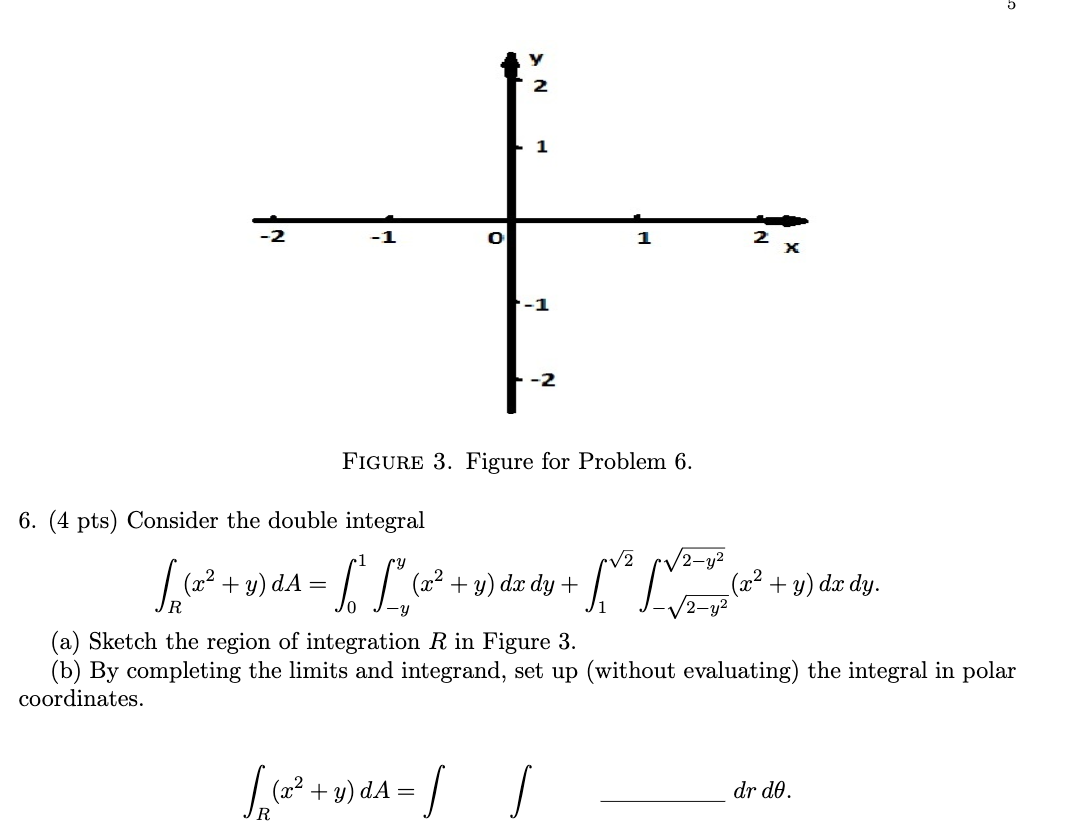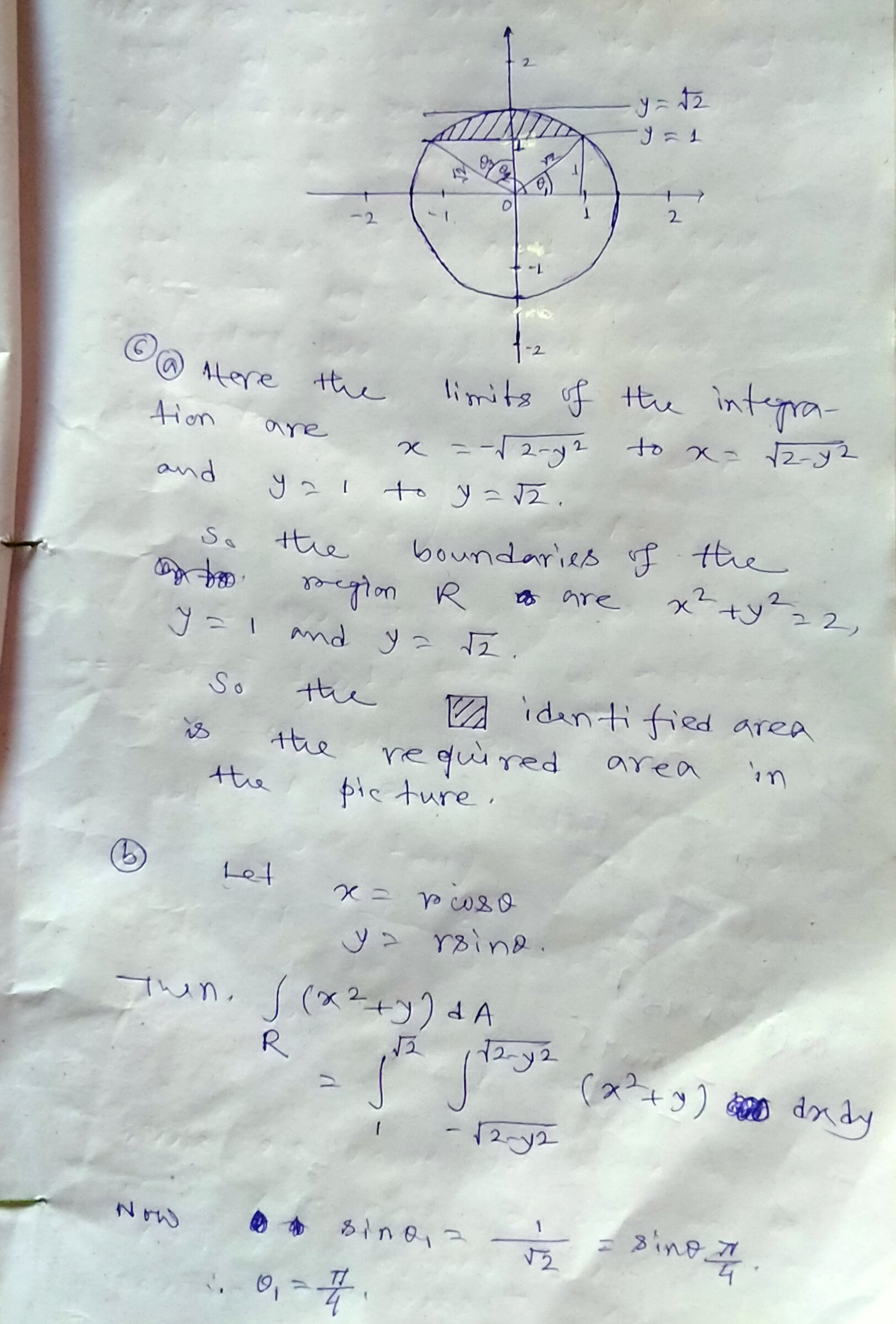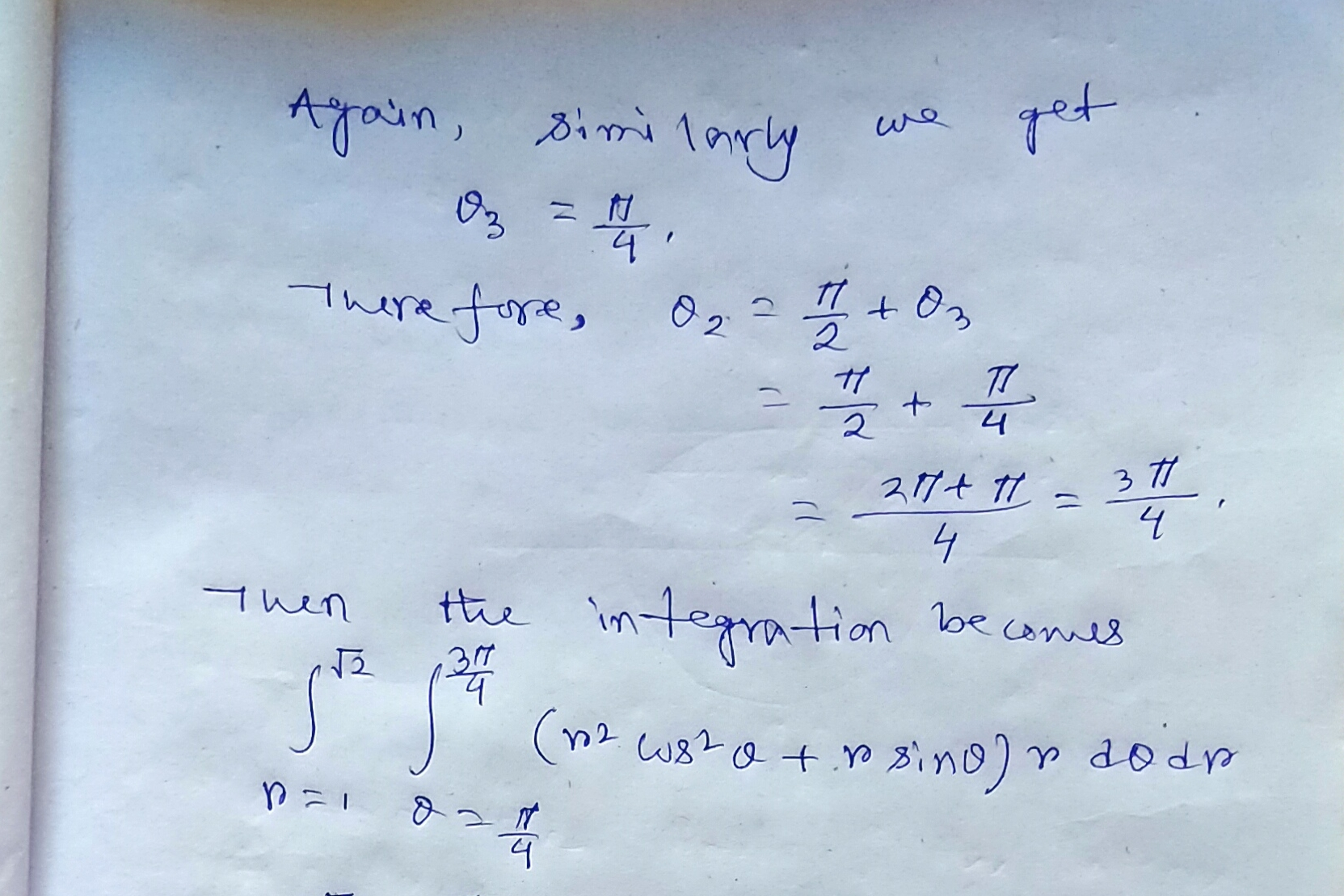#### Earn Coins

Coins can be redeemed for fabulous gifts.

Similar Homework Help Questions
• ### 6. (4 pts) Consider the double integral∫R(x2+y)dA=∫10∫y−y(x2+y)dxdy+∫√21∫√2−y2−√2−y2(x2+y)dxdy.(a) Sketch the region of integrationRin Figure 3.(b) By completing...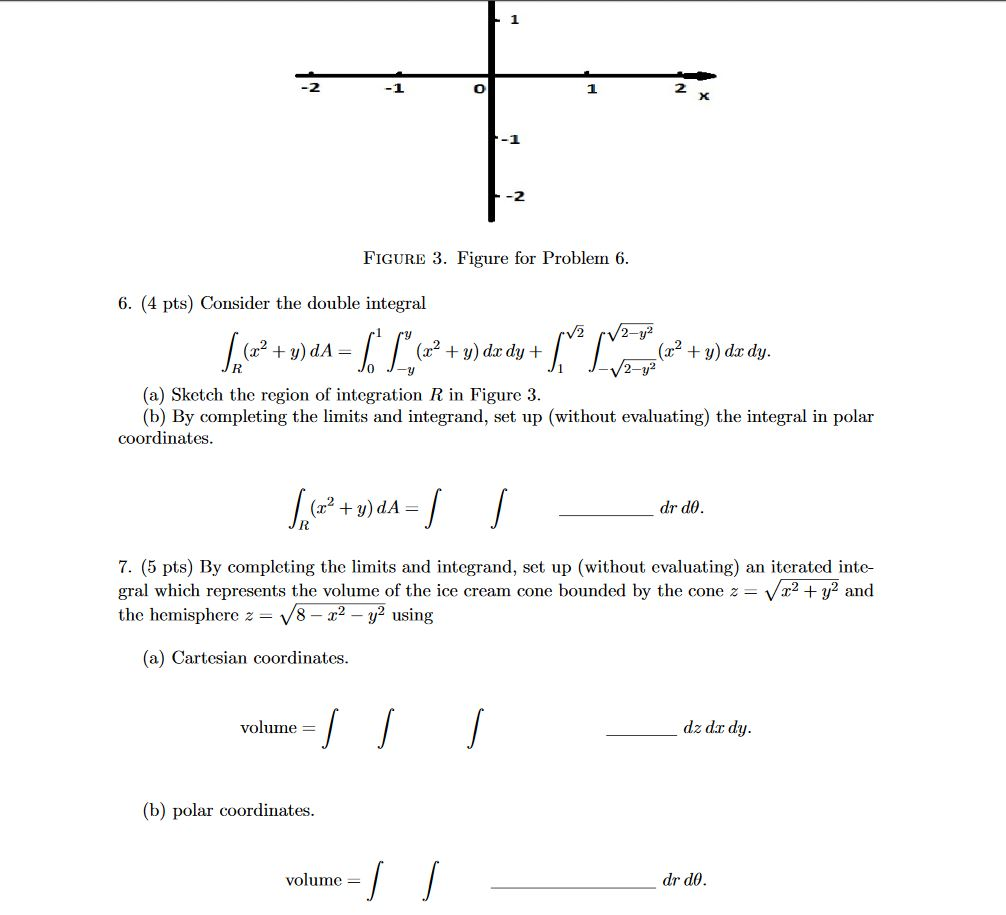6. (4 pts) Consider the double integral∫R(x2+y)dA=∫10∫y−y(x2+y)dxdy+∫√21∫√2−y2−√2−y2(x2+y)dxdy.(a) Sketch the region of integrationRin Figure 3.(b) By completing the limits and integrand, set up (without evaluating) the integral in polar coordinates. -1 -2 FIGURE 3. Figure for Problem 6. 6. (4 pts) Consider the double integral V2 /2-y² + = (x2 + y) dx dy + + y) do dy. 2-y2 (a) Sketch the region of integration R in Figure 3. (b) By completing the limits and integrand, set up (without evaluating)...

• ### 6. (4 pts) Consider the double integral∫R(x2+y)dA=∫10∫y−y(x2+y)dxdy+∫√21∫√2−y2−√2−y2(x2+y)dxdy.(a) Sketch the region of integration R in Figure 3.(b)...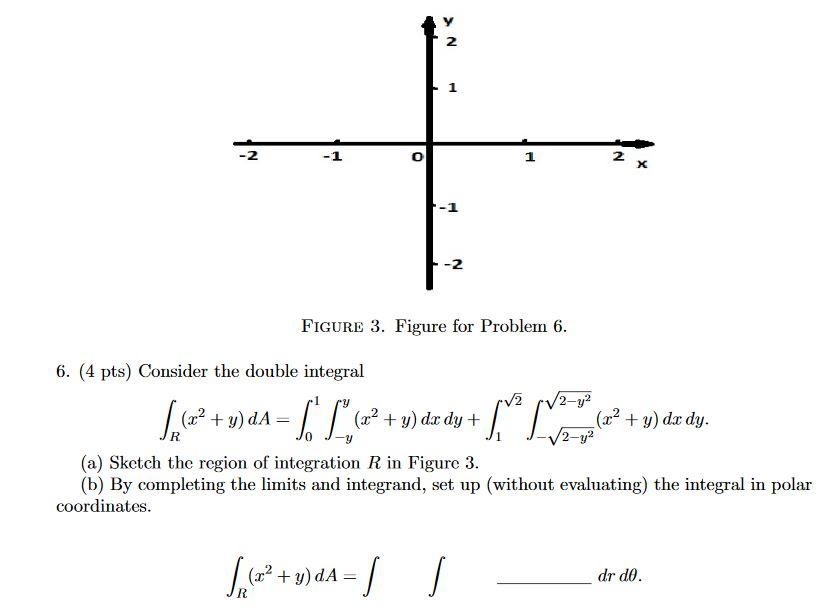6. (4 pts) Consider the double integral∫R(x2+y)dA=∫10∫y−y(x2+y)dxdy+∫√21∫√2−y2−√2−y2(x2+y)dxdy.(a) Sketch the region of integration R in Figure 3.(b) By completing the limits and integrand, set up (without evaluating) the integral in polar coordinates. 2 1 2 X -2 FIGURE 3. Figure for Problem 6. 6. (4 pts) Consider the double integral V2 2-y2 (2? + y) dA= (32 + y) dx dy + (x2 + y) dx dy. 2-y? (a) ketch the region of integration R in Figure 3. (b) By completing...

• ### 6. (4 pts) Consider the double integral∫R(x2+y)dA=∫10∫y−y(x2+y)dxdy+∫√21∫√2−y2−√2−y2(x2+y)dxdy.(a) Sketch the region of integration R in Figure 3.(b)...6. (4 pts) Consider the double integral∫R(x2+y)dA=∫10∫y−y(x2+y)dxdy+∫√21∫√2−y2−√2−y2(x2+y)dxdy.(a) Sketch the region of integration R in Figure 3.(b) By completing the limits and integrand, set up (without evaluating) the integral in polar coordinates.∫R(x2+y)dA=∫∫drdθ.7. (5 pts) By completing the limits and integrand, set up (without evaluating) an iterated inte-gral which represents the volume of the ice cream cone bounded by the cone z=√x2+y2andthe hemisphere z=√8−x2−y2using(a) Cartesian coordinates.volume =∫∫∫dz dxdy.(b) polar coordinates.volume =∫∫drdθ. -1 -2 FIGURE 3. Figure for Problem 6. 6. (4 pts)...

• ### 7. (5 pts) By completing the limits and integrand, set up (without evaluating) an iterated inte-...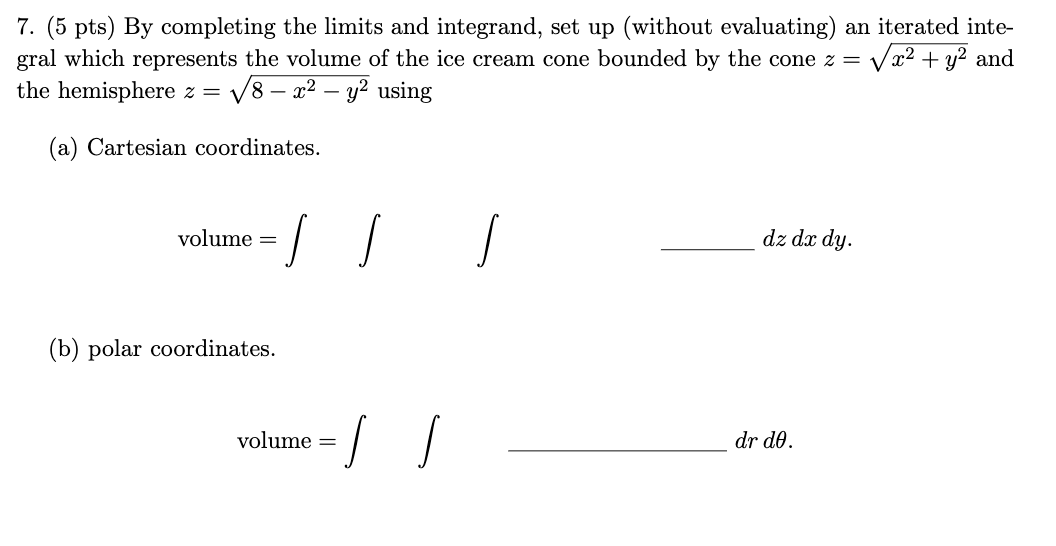7. (5 pts) By completing the limits and integrand, set up (without evaluating) an iterated inte- gral which represents the volume of the ice cream cone bounded by the cone z = V x2 + y2 and the hemisphere z = V8 – x2 - y2 using (a) Cartesian coordinates. volume = dz dx dy. (b) polar coordinates. volume = I dr de.

• ### 7. (5 pts) By completing the limits and integrand, set up (without evaluating) an iterated inte-gral...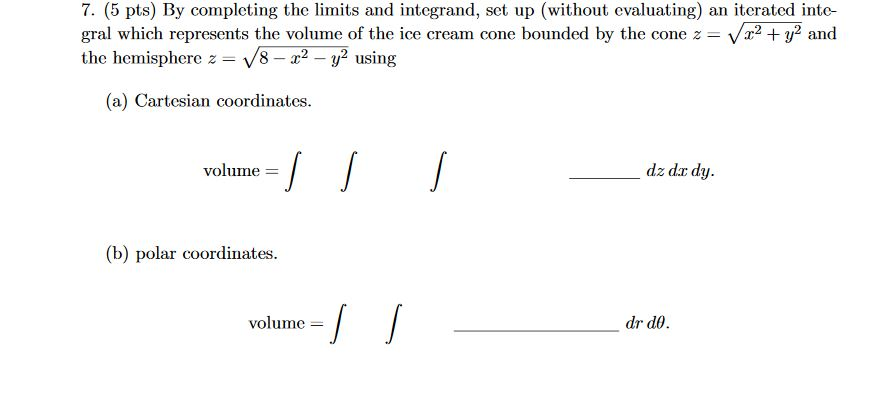7. (5 pts) By completing the limits and integrand, set up (without evaluating) an iterated inte-gral which represents the volume of the ice cream cone bounded by the cone z=√x2+y2andthe hemisphere z=√8−x2−y2using(a) Cartesian coordinates. 7. (5 pts) By completing the limits and integrand, set up (without evaluating) an iterated inte- gral which represents the volume of the ice cream cone bounded by the cone z = Vr2 + y2 and the hemisphere z = 18 - 22 - y2 using...

• ### 7. (5 pts) By completing the limits and integrand, set up (without evaluating) an iterated inte-...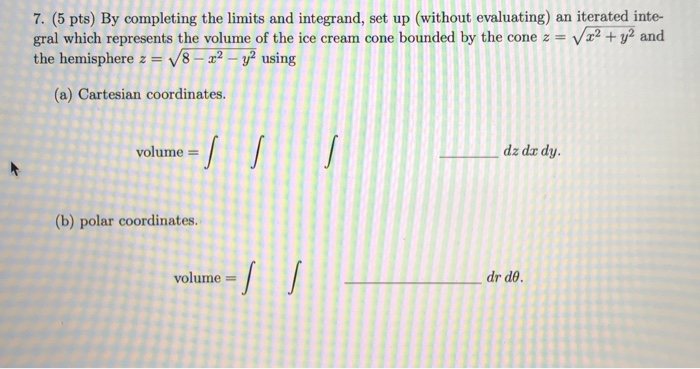7. (5 pts) By completing the limits and integrand, set up (without evaluating) an iterated inte- gral which represents the volume of the ice cream cone bounded by the cone z = V x2 + y2 and the hemisphere z = V8 - 22 - y2 using (a) Cartesian coordinates. volume = dz dar dy. (b) polar coordinates. volume = dr de.

• ### Assignment 7: Problem 9 Previous Next Problem List (1 point) Sketch the region of integration and evaluate by chan...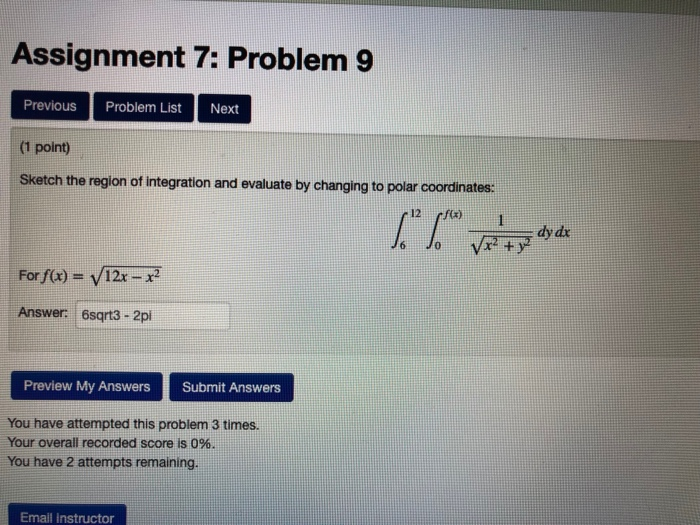Assignment 7: Problem 9 Previous Next Problem List (1 point) Sketch the region of integration and evaluate by changing to polar coordinates: 12 rf) 1 y dx V +y J6 For f(x) = V12x- Answer: 6sgrt3-2p Submit Answers Preview My Answers You have attempted this problem 3 times. Your overall recorded score is 0%6. You have 2 attempts remaining. Email Instructor Assignment 6: Problem 9 Problem List Next Previous (1 point) Set up a double integral in rectangular coordinates for...

• ### 16. o integrad [**** The triple da dy dz describes the solid pictured at right. Rewrite...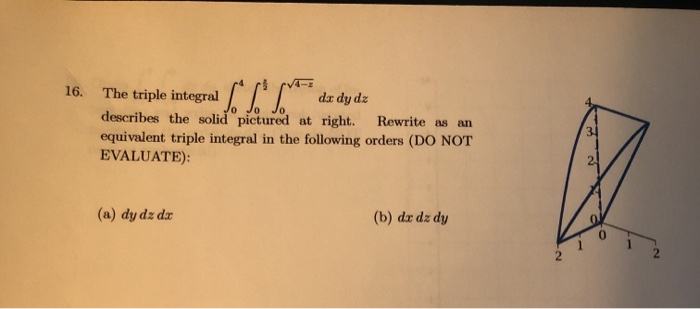16. o integrad [**** The triple da dy dz describes the solid pictured at right. Rewrite as an equivalent triple integral in the following orders (DO NOT EVALUATE): 31 (a) dy dz dx (b) du dz dy 2. 16-2 21. Given dy da, 16- (a) Sketch the region of integration and write an equivalent iterated integral in the order dx dy. (You do not need to evaluate it!) (b) Now write it as an equivalent iterated integral in polar coordinates....

• ### (a) Evaluate the double integral 4. (sin cos y) dy dr. Hint: You may need the formula for integration by parts (b) Show...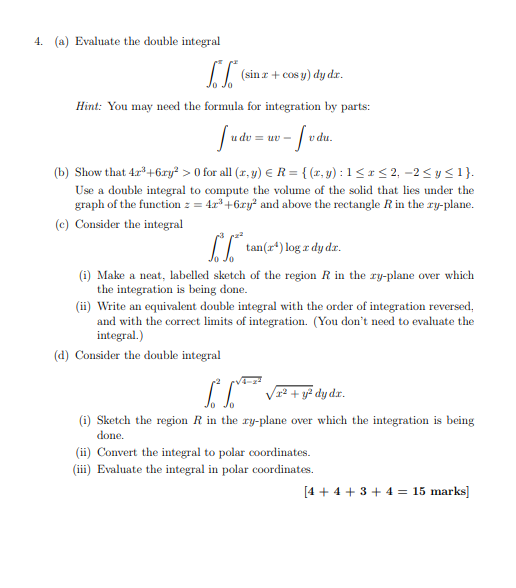(a) Evaluate the double integral 4. (sin cos y) dy dr. Hint: You may need the formula for integration by parts (b) Show that 4r+6ry>0 for all (r,y) ER-(x,y): 1S2,-2Sysi) Use a double integral to compute the volume of the solid that lies under the graph of the function 4+6ry and above the rectangle R in the ry-plane. e) Consider the integral tan(r) log a dyd. (i) Make a neat, labelled sketch of the region R in the ry-plane over...

• ### Thanks In evaluating a double integral over a region D, a sum of iterated integrals was...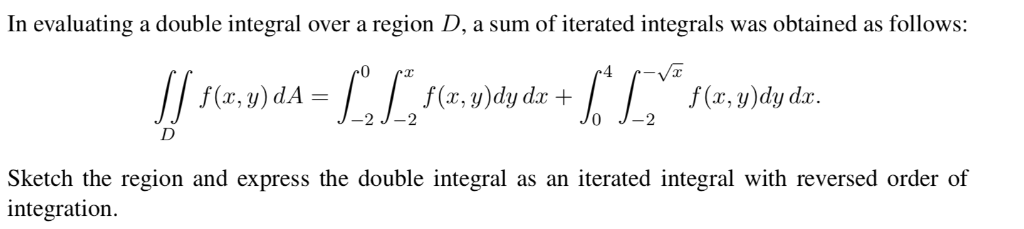Thanks In evaluating a double integral over a region D, a sum of iterated integrals was obtained as follows: 0 f(x, y)dy dr f (r, y)dy d f(x, y) dA -2 2 TJ= Sketch the region and express the double integral integration as an iterated integral with reversed order of